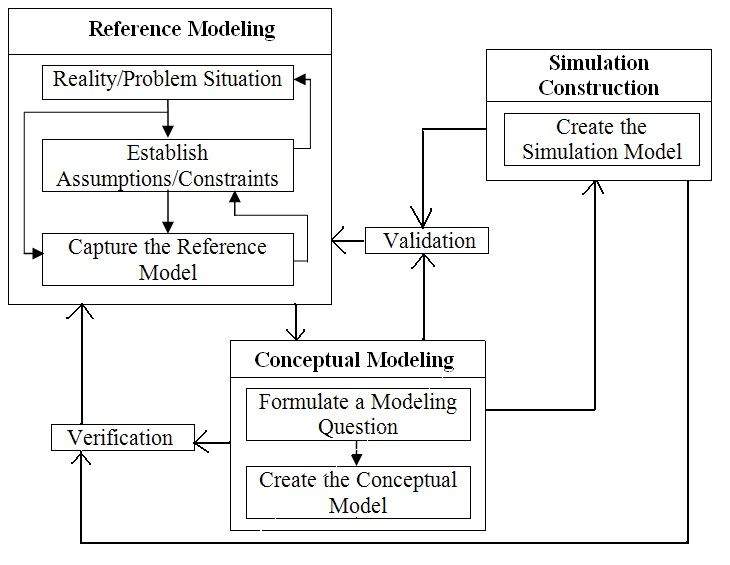# A Multi-Paradigm Modeling Framework for Modeling and Simulating Problem Situations

### Abstract

This paper proposes a multi-paradigm modeling framework (MPMF) for modeling and simulating problem situations (problems whose specification is not agreed upon). The MPMF allows for a different set of questions to be addressed from a problem situation than is possible through the use of a single modeling paradigm. The framework identifies different levels of granularity (macro, meso, and micro) from what is known and assumed about the problem situation. These levels of granularity are independently mapped to different modeling paradigms. These modeling paradigms are then combined to provide a comprehensive model and corresponding simulation of the problem situation. Finally, the MPMF is implemented to model and simulate the problem situation of representing the spread of obesity.Figure 1: Overview of the Modeling and Simulation – System Development Framework